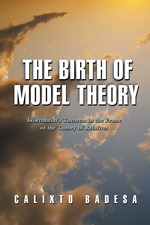Have library access? Log in through your library# The Birth of Model Theory: Lowenheim's Theorem in the Frame of the Theory of Relatives

CALIXTO BADESA
Translated by Michael Maudsley
Copyright Date: 2004
Pages: 256
https://www.jstor.org/stable/j.ctt7s4kb

## Table of Contents

1. Front Matter
(pp. i-vi)
2. Table of Contents
(pp. vii-viii)
3. Preface
(pp. ix-xiv)
4. Chapter One Algebra of Classes and Propositional Calculus
(pp. 1-30)

1.1.1 George Boole (1815–1864) is justly considered the founder of mathematical logic in the sense that he was the first to develop logic using mathematical techniques. Leibniz (1646–1716) had been aware of this possibility, and De Morgan (1806–1878) worked in the same direction, but Boole was the first to present logic as a mathematical theory, which he developed following the algebraic model. His most important contributions are found inThe mathematical analysis of logic, his first work on logic, andAn investigation of the laws of thought, which contains the fullest presentation of his ideas...

5. Chapter Two The Theory of Relatives
(pp. 31-72)

2.1.1 The study of the logic of relatives (or of relations) was begun by A. De Morgan in his 1859 paper “On the syllogism IV and on the logic of relations.” If Boole can be considered the founder of mathematical logic, De Morgan deserves acknowledgment as the first logician to appreciate the limitations of traditional logic and to recognize the importance to logic of the study of relations. De Morgan, for a number of reasons, did not establish the foundations of a theory of relations. According to Tarski (, p. 73), the creator of the theory of binary relations is...

6. Chapter Three Changing the Order of Quantifiers
(pp. 73-106)

3.1.1 Löwenheim begins the proof of his theorem by showing that every formula is logically equivalent to a formula which has a certain normal form. A formula is in (Löwenheim) normal form if it is in prenex form and every existential quantifier precedes every universal quantifier.¹ Obviously, the central step in obtaining the normal form of a formula involves moving the existential quantifiers in front of the universal quantifiers, preserving logical equivalence. Löwenheim takes this step by generalizing and applying a transformation introduced by Schröder in the third volume ofVorlesungen.

Schröder only considers the problem of changing the order...

7. Chapter Four The Löwenheim Normal Form
(pp. 107-128)

4.1.1 As I stated at the beginning of the previous chapter, the first part of Löwenheim’s proof consists in showing that every equation is equivalent to an equation that has a certain normal form. Löwenheim considers an equation to be in normal form if it has the form$\Sigma \Pi F = 0$, whereFis a quantifier-free formula, Σ represents a possibly empty string of existential quantifiers (either type ∑ or$\underline \sum$) and$\Pi$represents a possibly empty string of universal quantifiers (as a special case,$F = 0$is in normal form).

Löwenheim assumes in “Über Möglichkeiten” that all equations are in the form...

8. Chapter Five Preliminaries to Löwenheim’s Theorem
(pp. 129-142)

Löwenheim uses the word “index” to refer both to variables of the formal language and to elements of the domain. Bearing in mind the fact that no distinction was made at that time between object language and metalanguage, and also that the same letters are used as variables of the formal language and as metalinguistic variables ranging over elements of the domain, a certain degree of inaccuracy is understandable. If the ambiguity amounted to this alone, there would be no need for any comment, since today we make all these distinctions and we are often not as careful as we...

9. Chapter Six Löwenheim’s Theorem
(pp. 143-206)

6.1.1 Today, we use the nameLöwenheim-Skolem theoremto refer to all the theorems that guarantee that if a set of formulas has a model of a particular cardinality, it also has a model of some other cardinality. The first theorem in the series (leaving open the question of whether it is also the weakest) is the second of the theorems that Löwenheim proves in “Über Möglichkeiten;” it states:

If the domain is at least denumerably infinite, it is no longer the case that a first-order fleeing equation (Fluchtzählgleichung) is satisfied for arbitrary values of the relative coefficients. (“Über Möglichkeiten,”...

10. Appendix. First-Order Logic with Fleeing Indices
(pp. 207-226)
11. References
(pp. 227-236)
12. Index
(pp. 237-240)